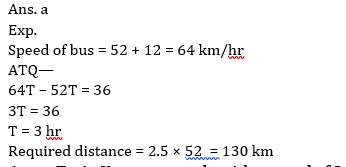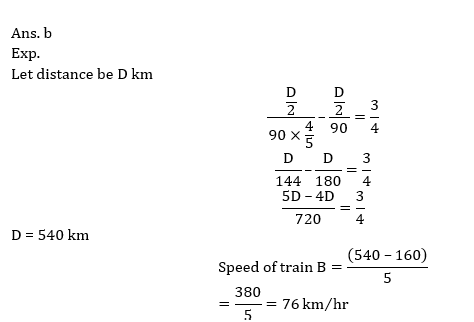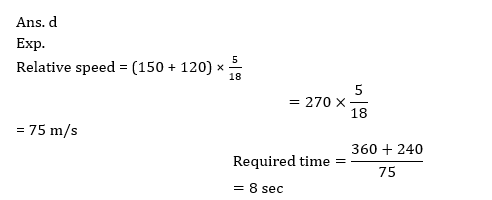# Speed Time & Distance for RRB PO & RRB CLERK : Quiz -10

## Speed Time & Distance for RRB PO & RRB CLERK: Quiz – 10

Speed Time and Distance is probably the most commonly asked Quantitative Aptitude question in various Banking Exams. Generally, 1-2 Time Speed and Distance Questions are asked in competitive examinations. Candidates must have a clear understanding of Time Speed and Distance Questions. So here we bring to you Time Speed and Distance Quiz in order to enhance your preparation. This Time Speed and Distance Quiz has a full explanation for each question. This Time Speed and Distance Quiz includes the latest or previous years’ question. This Time Speed and Distance Quiz is entirely free of cost.

1. Kim and Om are travelling from point A to B, which are 400 km apart. Travelling at a certain speed Kim takes one hour more than Om to reach point B. If Kim doubles her speed, she will take 1 hour 30 min less than Om to reach point B. At what speed was Om driving from point A to B? (in km/h)
(a) 90 km/h
(b) 70 km/h
(c) 160 km/h
(d) 80 km/h
(e) 100 km/h2. Two cars A and B are at point P and Q respectively and are at a distance of 250 km. They started to move towards each other and meet at point C after 2 hours. It is found that when car A reached point Q, car B after reaching point P, travelled 100 km more. Find the speed of car A.
(a) 310/7 km/hr
(b) 128/3 km/hr
(c) 625/12 km/hr
(d) 101/2 km/hr
(e) 152/3 km/hr3. Two trains P and Q started from two points A and B towards each other to cover 300 km distance. Speed of train Q is twice of speed of train P. If train P runs at its normal speed and train Q reduces its speed to 50% then it will take 2.5 hrs. more to meet each other. Find the time (in sec) in which train P can cover a platform of 150m length if the length of train P is given as 50m.
(a) 18 seconds
(b) 36 seconds
(c) 72 seconds
(d) 54 seconds
(e) None of these4. A train P with speed 64 km/hr crosses a pole in 27 second and an another train Q whose speed is 25/2 % less than train P crosses a man in 36 second. If both train move in opposite direction to each other, then find in how much time will they cross each other?
(a) 28.2 sec
(b) 36.2 sec
(c) 31.2 sec
(d) 38.2sec
(e) 39.2 sec5. A car travel 36 km less than a bus in T hours. Speed of bus is 12 km/hr more than speed of car, if speed of car is 52 km/hr. Find how much distance will be covered by car in (T- ½) hours?
(a) 130 km
(b) 140 km
(c) 145 km
(d) 135 km
(e) 125 km6. Train X crosses a pole with a speed of 24 m/s in ‘t’ seconds. Train Y crosses a platform of 60 m length in ‘2t’ seconds with a speed of 30 m/s. if Length of train Y is twice then that of train X. Find the length of train Y?
(a) 60 m
(b) 120 m
(c) 240 m
(d) 90 m
(e) 150 m7. Train A travelling with the speed of 90 km/hr and after covered half of a distance train lost its speed by 1/5 th and reached station late by 45 minutes. In 5 hour Train B travel 160 km less than the above distance travel by Train A then find the speed of train A ?
(a) 72km/hr
(b) 76km/hr
(c) 68km/hr
(d) 64km/hr
(e) 60km/hr8. Two trains of lengths 360 m and 240 m are running in the opposite direction with speed of 120 kmph and 150 kmph respectively. The time taken by them to cross each other is ?
(a) 10sec
(b) 6 sec
(c) 4sec
(d) 8 sec
(e) 2 sec9. A car covers distance of 360 km in three equal parts with three different speeds in total 7.5 hours. If car covered first part with some speed ‘s’, second part with 20% increased speed than the first and third part with 25% increased speed than the second. Find the speed at which car covered third part of journey?
(a) 56km/hr
(b) 36km/hr
(c) 40km/hr
(d) 60km/hr
(e) 48 km/hr10. Train A moving with 50 m/s crosses a man sitting in another train B moving in opposite direction in 4 sec. If length of train B is 20% more than length of train A and it crosses a pole in 24 sec. Find length of train A ?
(a) 140 m
(b) 320 m
(c) 160 m
(d) 250 m
(e) 220 m#### Attempt Quantitative Aptitude Topic Wise Online Test Series

Recommended PDF’s for :

#### Most important PDF’s for Bank, SSC, Railway and Other Government Exam : Download PDF Now

AATMA-NIRBHAR Series- Static GK/Awareness Practice Ebook PDF Get PDF here
The Banking Awareness 500 MCQs E-book| Bilingual (Hindi + English) Get PDF here
AATMA-NIRBHAR Series- Banking Awareness Practice Ebook PDF Get PDF here
Computer Awareness Capsule 2.O Get PDF here
AATMA-NIRBHAR Series Quantitative Aptitude Topic-Wise PDF Get PDF here
AATMA-NIRBHAR Series Reasoning Topic-Wise PDF Get PDF Here
Memory Based Puzzle E-book | 2016-19 Exams Covered Get PDF here
Caselet Data Interpretation 200 Questions Get PDF here
Puzzle & Seating Arrangement E-Book for BANK PO MAINS (Vol-1) Get PDF here
ARITHMETIC DATA INTERPRETATION 2.O E-book Get PDF here
3